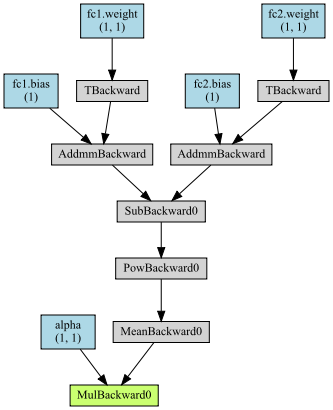# Cannot calculate second order gradients even though `create_graph=True`

I have a training loop iteration that looks like this…

``````mu, logvar = m(x)

loss = alpha * criterion(mu, logvar)

loss.backward(retain_graph=True, create_graph=True)
for p in m.parameters():
p = p - LR * p.grad

x_next, y_next = data[j + 1]
mu_next, logvar_next = m(x_next)

meta_loss = criterion(mu_next, logvar_next)
``````

and pytorch is complaining that `RuntimeError: One of the differentiated Tensors appears to not have been used in the graph. Set allow_unused=True if this is the desired behavior.` but as far as I can tell it should have been used in the graph because it was multiplied by the loss and then the graph should have persisted, and then when calling the model again I should be able to get the gradients with respect to the original `alpha`.

Where have I gone wrong?

The computation graph, where `alpha` was used seems to be unrelated to the second computation graph, as you are using new input tensors and I cannot see the connection between these graphs.

Shouldn’t the connection be the model parameters themselves?

If `alpha` was created through the previous graph, then yes.
Otherwise, if `alpha` is a leaf node, then it won’t connect the computation graphs.

@ptrblck thanks for the responses. Maybe I am just dense, but I am not understanding what you said. I’ve been through this stuff many times in the docs but I still don’t get exactly why this isn’t working. I have updated my example to be more simple and complete.

Does my updated example look like it would work? Why or why not? Thanks again

``````class MyModel(nn.Module):
def __init__(self):
super(MyModel, self).__init__()
self.fc1 = nn.Linear(1, 1)
self.fc2 = nn.Linear(1, 1)

def forward(self, x):
mu = self.fc1(x)
logvar = self.fc2(x)
return mu, logvar

# Setup
m = MyModel()
criterion = nn.MSELoss()
x = torch.randn(1, 1)
LR = 1.

# First graph creation
mu, logvar = m(x)

print(alpha.is_leaf) # alpha is a leaf variable
loss = alpha * criterion(mu, logvar) # alpha is now used in the computation graph via the multiplication

loss.backward(retain_graph=True, create_graph=True)
for p in m.parameters():
p = p - LR * p.grad

# Next graph
x_next = torch.randn(1, 1)
mu_next, logvar_next = m(x_next) # this creates a new computation graph
# no outputs from the first computation were used here

meta_loss = criterion(mu_next, logvar_next) # loss calculated for the new graph
# calculate sum of grads of meta_loss w.r.t. alpha,
# but alpha wasn't used in the creation of meta loss!
``````

The main issue is, that you are trying to calculate the gradients of `meta_loss` w.r.t. `alpha`, but when you write down the formula, how `meta_loss` was computed, you won’t find `alpha` in there.

ok, that is very clear now…but… alpha was used in the calculation of the parameters of the model before `m(x_next)` was called. I’m assuming that the graph containing this information was either never created or destroyed?

The graph was created and `loss` is attached to it.
Using `torchviz` you would get this graph for the first forward pass with `alpha` included:and this graph for the second part:As you can see, `alpha` is in the graph in the last multiplication.
However, these graphs are not depending on each other, as they were created in two separate forward passes and new input.

1 Like

right. that is a very helpful visualization. I see what is happening and it is very clear to me that the gradients don’t make it past the backward call. I still don’t see why they dont propagate through the parameter update though.

• I set `retain_graph` and `create_graph`
• I manually update the parameters (without calling `no_grad`) and I also don’t use the in-place operations that are in the optimizers which avoid the differentiation graph (AFAIK)

This chain of events leads me to believe that the graph should still be stored and the top graph you posted and the bottom graph should be connected from `MeanBackward0` to `TBackward` with the inputs coming in separately.

This is a common pattern in meta learning if I understand it correctly and I don’t see why the graphs should be separated given my bullet points above.

I think your bullet points are correct.
Also,

would be true.

However, `MeanBackward0` and `TBackward` will not connect the graphs.

If these graphs would be connected, then also this dummy code:

``````lin = nn.Linear(1, 1)

x1 = torch.randn(1, 1)
out1 = lin(x1)

x2 = torch.randn(1, 1)
out2 = lin(x2)
``````

would create a single graph, which is not the case.

I have the feeling, that I’m missing something, as I’m not deeply familiar with meta learning.

CC @albanD to see what I’m missing and correct me.

1 Like

The method in the following two papers, as well as the corresponding github link use second order gradients in this way.

I think the torchmeta library in this link is doing something in the background to allow this to happen, but I can’t see where the disconnect is and why my own code never seems to recognize the second order gradients when I think it should

I think the problem in your code is:

``````for p in m.parameters():
p = p - LR * p.grad
``````

This does not modify `p` inplace ! It just assigns the result to a variable name p that you override just after. So you could remove these lines and your code would run the same.
This is why you don’t see the link.

Another issue you’re gonna face is that `nn.Parameter()` are built explicitly to be leaf Tensors (with no history) and so you won’t be able to change them with a Tensor you try to backprop through.

You can check the higher package to do this properly (they solve this problem of nn.Parameter for you). Otherwise, if you want to do it manually, you can find some info in this thread: How does one have the parameters of a model NOT BE LEAFS?

@albanD @ptrblck thanks for taking the time to clarify this